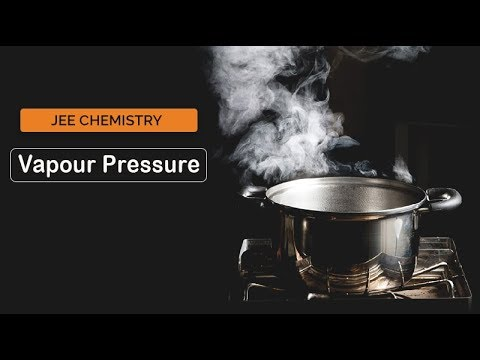Checkout JEE MAINS 2022 Question Paper Analysis : Checkout JEE MAINS 2022 Question Paper Analysis :

# Ideal Solution

## What is an Ideal Solution?

An ideal solution is a mixture in which the molecules of different species are distinguishable, however, unlike the ideal gas, the molecules in ideal solution exert forces on one another. When those forces are the same for all molecules independent of species then a solution is said to be ideal.

If we take the simplest definition of an ideal solution, then it is described as a homogeneous solution where the interaction between molecules of components (solute and solvents) is exactly the same to the interactions between the molecules of each component itself. These types of solutions follow Raoult’s Law at almost all levels of concentration and temperatures.

## Recommended Videos## Characteristics of Ideal Solution

An ideal solution can be obtained by mixing a solute and a solvent which consist of similar molecular structure and size. If we take two substances X and Y and mix them we will see that there are several intermolecular forces that exist between them.

For example,

• X and X experience intermolecular forces of attraction.
• Y and Y experience intermolecular forces of attraction.
• X and Y experience intramolecular forces of attraction.

Here, when the intermolecular forces of attraction are the same or equal, it gives us an ideal solution.

Understanding the concept of an ideal solution is really important, especially when we are studying chemical thermodynamics and topics like colligative properties.

## Raoult’s Law

Raoult’s law states that “the relative lowering of the vapour pressure of a solvent by dissolving a non-volatile electrolyte is equal to the mole fraction of the solute”.

Thus ΔP/PAo = No. of moles of solute/ Total moles of solute and solvent

Thus

$$\begin{array}{l}\frac{ΔP}{PA}\end{array}$$
=  PoA –
$$\begin{array}{l}\frac{PA}{PoA} = XB = \frac{n}{n+N} \end{array}$$

ΔP/PA = PoA – /PoA = XB = n/n+N

Where n and N are the numbers of moles of solute and solvent respectively.

In a dilute solution
N>>n

Hence
XB = n/N = w/m/W/M
Where w and W are the weights of solute and solvent and m and M are their molecular weights respectively.

Therefore,

PoA – PA/PoA = P/PoA = wM/Wm

## Properties of an Ideal Solution

An ideal solution most of the time has physical properties that are closely related to the properties of the pure components. Some of its properties are;

• The enthalpy of solution is zero. If the enthalpy of the solution gets closer to zero it is more likely to show an ideal behaviour.
$$\begin{array}{l}\Delta _{mix} H = 0\end{array}$$
• The volume of mixing is also zero.
$$\begin{array}{l}\Delta _{mix} V = 0\end{array}$$

## Examples of Ideal Solution

Getting a well-balanced ideal solution can be rare but some solutions can sometimes exhibit ideal behaviour. Some examples include;

• Toluene and Benzene.
• Ethyl Iodide and Ethyl Bromide.
• Bromobenzene and Chlorobenzene.
• N-heptane and n-hexane.

Notably, when we talk about the concentrations of the solutions most of the dilute solutions also have or show characteristics of an ideal solution.

## FAQs

1. What do you mean by an ideal solution?
Ans: A solution where the interaction of component molecules does not vary from the interactions of each component’s molecules. The ideal solutions are those at all concentrations and temperatures that obey Raoult’s law.

2. What are the characteristics of an ideal solution?
Ans: n ideal solution has the following characteristics: (i) mixing volume change should be zero. (ii) mixing heat change should be zero.

3. What is Raoult’s Law formula?
Ans: Raoult’s law is a chemical law that refers to a solution’s vapour pressure based on the mole fraction of a solution that is applied. Raoult’s Law is expressed by the formula, Resolution = Χsolvent x Psolvent

4. What does Raoult’s law state?
Ans: Raoult’s law states that the solution’s vapour pressure is equal to the sum of each volatile component’s vapour pressure if it was strictly multiplied by the mole fraction of that component in the solution.

5. What makes an ideal gas?
Ans: An ideal gas is defined as one where all collisions between atoms or molecules are perfectly elastic and where there are no attractive intermolecular forces. One can imagine it as a series of colliding perfectly hard spheres, but not communicating with each other.

Test your Knowledge on Ideal solution!